﻿ 第二类Hammerstein积分方程的一种新解法 A New Method for Solving a Hammerstein Integral Equation of the Second Kind

Vol.05 No.02(2016), Article ID:17528,8 pages
10.12677/AAM.2016.52025

A New Method for Solving a Hammerstein Integral Equation of the Second Kind

Fangfang Wu, Xianci Zhong, Qiongao Huang

School of Mathematics and Information Science, Guangxi University, Nanning Guangxi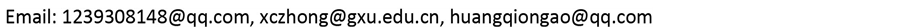Received: Apr. 21st, 2016; accepted: May 9th, 2016; published May 12th, 2016ABSTRACT

A nonlinear Hammerstein integral equation of the second kind is investigated in the present paper. The uniqueness of solution is considered by using the fixed point theorem in Banach spaces. A new numerical method is proposed by using the integral mean value theorem and the idea of piecewise approximation. An approximate solution is made and its convergence and error estimate are further analyzed. Numerical results are carried out to verify the feasibility and novelty of the proposed solution procedures.

Keywords:Hammerstein Integral Equation of the Second Kind, Approximate Solution, Convergence and Error Estimate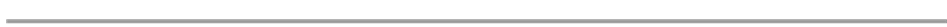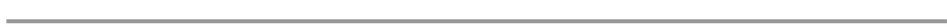1. 引言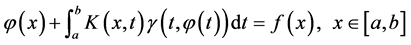(1)

2. 新的数值方法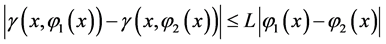(2)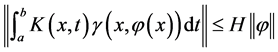(3)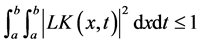(4)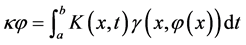(5)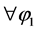，应用Lipschitz条件和Cauchy-Schwarz不等式有：(6)(7)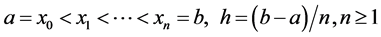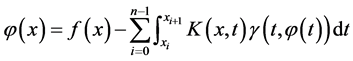(8)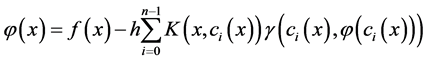(9)(10)(11)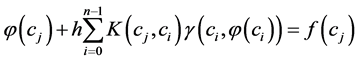(12)(13)(14)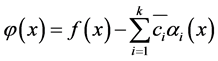(15)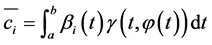(16)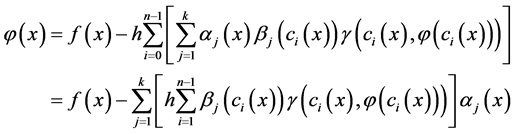(17)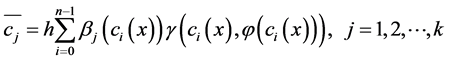(18)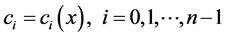(19)(20)

3. 近似解的收敛性和误差分析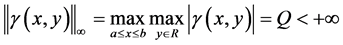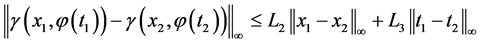(22)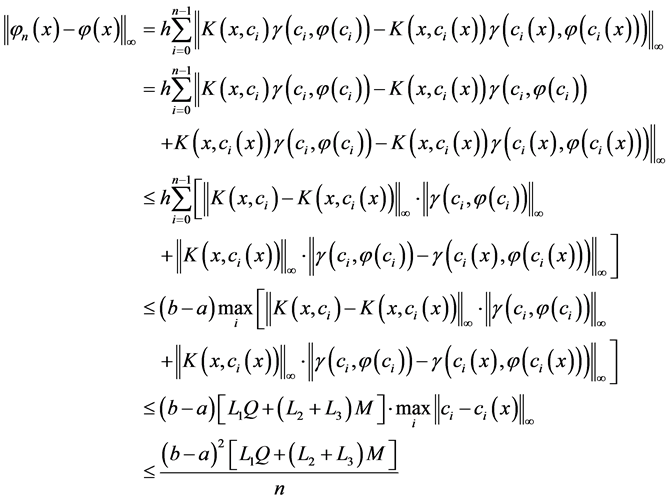(23)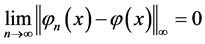(24)

4. 数值实例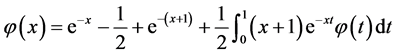Table 1. The absolute errors of approximate and exact solutions for Example 1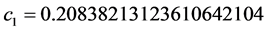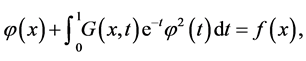Table 2. The absolute errors of approximate and exact solutions for Example 3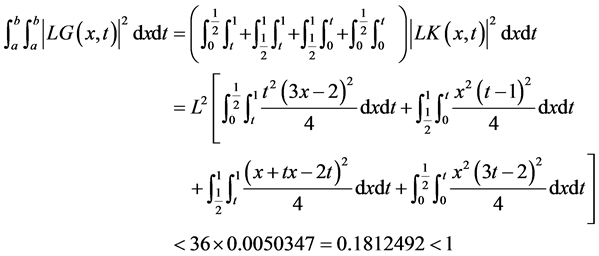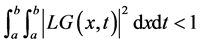，所以根据定理1可知该非线性三点边值问题有唯一解。使用本文的新方法求解此第二类Hammerstein积分方程，这里我们选择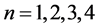分别进行计算，得到的近似解和准确解的绝对误差如上表2所示。从表格2中我们可以看到随着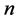的增大，近似解和准确解的绝对误差在逐渐减小，所得结果很好的符合了定理2的分析。

5. 结语

A New Method for Solving a Hammerstein Integral Equation of the Second Kind[J]. 应用数学进展, 2016, 05(02): 184-191. http://dx.doi.org/10.12677/AAM.2016.52025

1. 1. Keller, J.B. and Olmstead, W.E. (1972) Temperature of Nonlinearly Radiating Semi-Infinite Solid. Quarterly of Applied Mathematics, 29, 559-566.

2. 2. Olmstead, W.E. and Handelsman, R.A. (1996) Diffusion in a Semi-Infinite Region with Nonlinear Surface Dissipation. SIAM Review, 18, 275-291. http://dx.doi.org/10.1137/1018044

3. 3. Abdou, M.A. and Badr, A.A. (2002) On a Method for Solving an Integral Equation in the Displacement Contact Problem. Journal of Mathematical Analysis and Applications, 127, 65-78. http://dx.doi.org/10.1016/s0096-3003(01)00003-0

4. 4. Hashemizadeha, E. and Rostami, M. (2015) Numerical Solution of Hammerstein Integral Equations of Mixed Type Using the Sinc-Collocation Method. Journal of Mathe-matical Analysis and Applications, 279, 31-39.

5. 5. Kaneko, H. and Xu, Y.S. (1991) Degenerate Kernel Method for Hammerstein Equation. Mathematics of Computation, 56, 141-148. http://dx.doi.org/10.1090/S0025-5718-1991-1052097-9

6. 6. Abdoua, M.A., El-Boraib, M.M. and El-Kojokb, M.M. (2009) Toeplitz Matrix Method and Nonlinear Integral Equation of Hammerstein Type. Journal of Mathematical Analysis and Applications, 223, 765-776. http://dx.doi.org/10.1016/j.cam.2008.02.012

7. 7. Atkinson, K.E. (1997) The Numerical Solution of Integral Equ-ations of the Second Kind. Cambridge University Press, Cambridge. http://dx.doi.org/10.1017/CBO9780511626340

8. 8. 路见可, 钟寿国. 积分方程论[M]. 北京: 高教出版社, 1990.

9. 9. Zhong, X.C. (2013) A New Nystrom-Type Method for Fredholm Integral Equations of the Second Kind. Applied Mathematics and Computation, 219, 8842-8847. http://dx.doi.org/10.1016/j.amc.2013.03.036

10. 10. Kress, R. (1998) Numerical Analysis. Springer-Verlag, Berlin. http://dx.doi.org/10.1007/978-1-4612-0599-9

11. 11. Maleknejad, K. and Nedaiasl, K. (2011) Application of Sinc-Collocation Method for Solving a Class of Nonlinear Fredholm Integral Equations. Computers & Mathematics with Applications, 62, 3292-3303. http://dx.doi.org/10.1016/j.camwa.2011.08.045

12. 12. Borzabadi, A.H., Kamyad, A.V. and Mehne, H.H. (2006) A Different Approach for Solving the Nonlinear Fredholm Integral Equations of the Second Kind. Applied Mathematics and Computation, 173, 724-735. http://dx.doi.org/10.1016/j.amc.2005.04.008

13. 13. Borzabadi, A.H. and Fard, O.S. (2009) A Numerical Scheme for a Class of Nonlinear Fredholm Integral Equations of the Second Kind. Journal of Mathematical Analysis and Ap-plications, 232, 449-454. http://dx.doi.org/10.1016/j.cam.2009.06.038